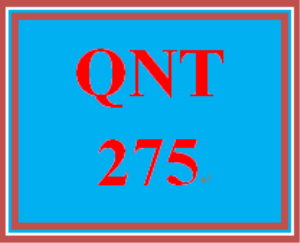QNT 275 Week 3 Practice: Connect Knowledge Check

PLDZ-7497 In Stock
\$ 7.00 USD
Description

https://uopcourses.com/category/qnt-275/

More courses: https://uopcourses.com/

QNT 275 Week 3 Practice: Connect Knowledge Check
1.

A plant manager knows that the number of boxes of supplies received weekly is normally distributed with a mean of 200 and a standard deviation of 20. What percentage of the time will fewer than 160 boxes of supplies arrive in a week?

2.28%

4.56%

42.07%

57.93%

P(x < 160) = P(z < (160 − 200)/20) = P(z < −2) = 0.0228

2.

The z value tells us the number of standard deviations that a value x is from the mean.

True

False

3.

An event is a collection of sample space outcomes.

True

False

4.

Determine whether these two events are mutually exclusive: consumer with an unlisted phone number and a consumer who does not drive.

not mutually exclusive

mutually exclusive

5.

Determine whether these two events are mutually exclusive: unmarried person and a person with an employed spouse.

not mutually exclusive

mutually exclusive

6.

Which of the following statements about the binomial distribution is not correct?

Each trial results in a success or failure.

Trials are independent of each other.

The experiment consists of n identical trials.

The random variable of interest is continuous.

The probability of success remains constant from trial to trial.

7.

For a continuous random variable x, the height of the probability curve f(x) at a particular point indicates the value of the probability for that value.

True

False

8.

An important part of the customer service responsibilities of a cable company is the speed with which trouble in service can be repaired. Historically, the data show that the likelihood is 0.75 that troubles in a residential service can be repaired on the same day. For the first five troubles reported on a given day, what is the probability that all five will be repaired on the same day?

.2373

.9990

.0010

.6328

P(x = 5) = .2373

9.

A standard normal distribution has a mean of ____________ and standard deviation of ____________.

zero, one

zero, zero

one, zero

one, one

10.

A letter is drawn from the alphabet of 26 letters. What is the probability that the letter drawn is a vowel?

5/26

21/26

1/26

4/26

AEIOU; 5 vowels out of 26 letters.

11.

The set of all possible outcomes for an experiment is called a(n) ____________.

event

probability

sample space

experiment

12.

Using the following probability distribution table of the random variable x, what is the probability of x = 3?

5/15

2/15

1/15

3/15

All values of P(X) need to sum to 1, so 5/15 + 4/15 + 1/15 = 10/15 means P(X = 3) = 5/15.

Recent Reviews Write a Review
0 0 0 0 reviews